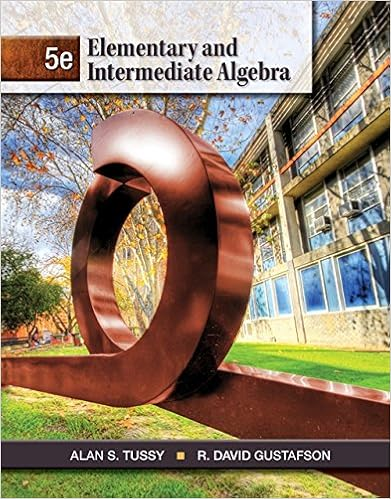# Can you reject the null hypothesis practice final 14

• Test Prep
• 14

This preview shows page 13 - 14 out of 14 pages.

##### We have textbook solutions for you!
The document you are viewing contains questions related to this textbook.The document you are viewing contains questions related to this textbook.
Chapter 1 / Exercise 29
Elementary and Intermediate Algebra
Tussy/GustafsonExpert Verified
Can you reject the null hypothesis?
##### We have textbook solutions for you!
The document you are viewing contains questions related to this textbook.The document you are viewing contains questions related to this textbook.
Chapter 1 / Exercise 29
Elementary and Intermediate Algebra
Tussy/GustafsonExpert Verified
Practice Final 14 8. Basic questions about statistical inference 8.1 The central limit theorem in statistics is used to make inferences about
8.2 P-values in hypothesis tests for a sample proportion are based on
8.3 When we say a sample estimate is “unbiased” this means
8.4 The variance of a sample estimate is a. Always independent of the expected value of the sample estimate b. Never independent of the expected value of the sample estimate c. Equal to the variance of the population parameter d. The square root of the standard deviation of the sample estimate e. None of the above
8.5 The assumption that the observations are “independent and identically distributed” in a linear regression, y i = 0 + 1 x i + pertains to i , i 1
8.6 The power of a test is the complement of the error rate when:
8.7 A p-value represents a. The probability that your results are correct
•••